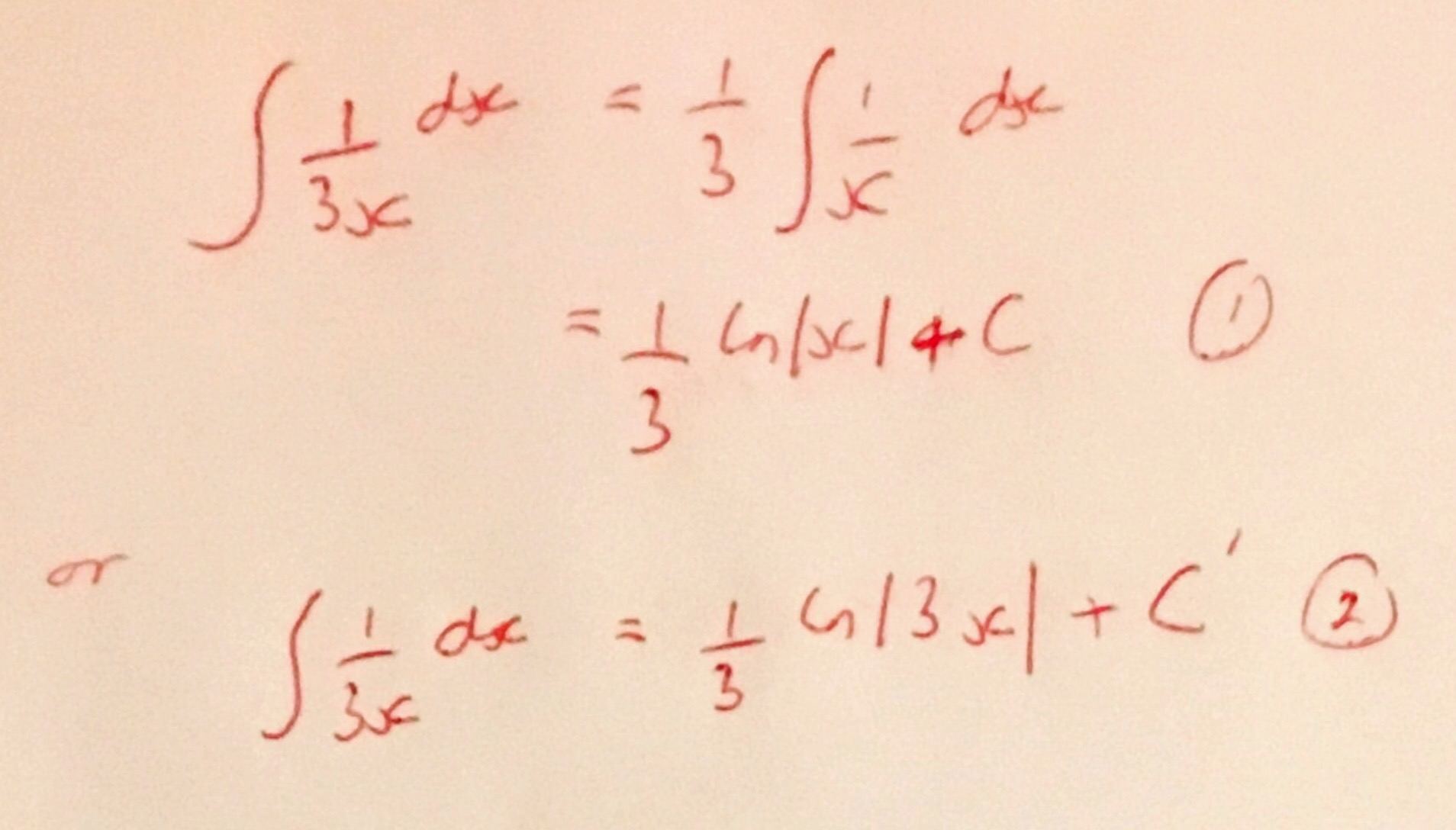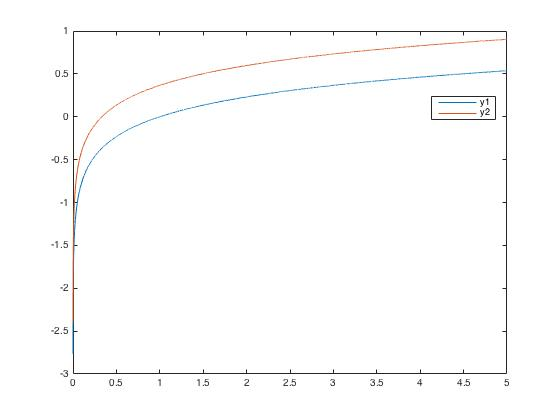Categories

# Manipulating Constants of Integration

I was recently asked about the integration of $$\frac{1}{3x}$$, this had come up in the solution of a differential equation. More specifically the fact that there are multiple ways to integrate this:At first glance these do not appear to be the same, however plotting them suggests that they are part of the same family of solution curves.Indeed a simple manipulation of the constant of integration shows them to be the same functions apart from the addition of a constant.Of course these constants would be fixed by an initial condition, and so both versions of the integration would lead to the same solution of the differential equation.

I have a vague memory of having this confusion myself when I was an A-Level student, but since then I haven’t really thought about this as I always “pull any constants outside of the integral” before integrating.

Thinking about this a bit more, I think that a great question for students would be to ask them to explain why $$[(1/3)\ln (x)]_1^3 = [(1/3)\ln (3x)]_1^3$$. I’m sure many would just evaluate the integrals as shown below, but asking for an explanation could lead to a deep discussion about the nature of the logarithm function.